# Markov switching dynamic regression models¶

This notebook provides an example of the use of Markov switching models in statsmodels to estimate dynamic regression models with changes in regime. It follows the examples in the Stata Markov switching documentation, which can be found at http://www.stata.com/manuals14/tsmswitch.pdf.

:

%matplotlib inline

import numpy as np
import pandas as pd
import statsmodels.api as sm
import matplotlib.pyplot as plt

# NBER recessions
from datetime import datetime

"USREC", "fred", start=datetime(1947, 1, 1), end=datetime(2013, 4, 1)
)


## Federal funds rate with switching intercept¶

The first example models the federal funds rate as noise around a constant intercept, but where the intercept changes during different regimes. The model is simply:

$r_t = \mu_{S_t} + \varepsilon_t \qquad \varepsilon_t \sim N(0, \sigma^2)$

where $$S_t \in \{0, 1\}$$, and the regime transitions according to

$\begin{split} P(S_t = s_t | S_{t-1} = s_{t-1}) = \begin{bmatrix} p_{00} & p_{10} \\ 1 - p_{00} & 1 - p_{10} \end{bmatrix}\end{split}$

We will estimate the parameters of this model by maximum likelihood: $$p_{00}, p_{10}, \mu_0, \mu_1, \sigma^2$$.

The data used in this example can be found at https://www.stata-press.com/data/r14/usmacro.

:

# Get the federal funds rate data
from statsmodels.tsa.regime_switching.tests.test_markov_regression import fedfunds

dta_fedfunds = pd.Series(
fedfunds, index=pd.date_range("1954-07-01", "2010-10-01", freq="QS")
)

# Plot the data
dta_fedfunds.plot(title="Federal funds rate", figsize=(12, 3))

# Fit the model
# (a switching mean is the default of the MarkovRegession model)
mod_fedfunds = sm.tsa.MarkovRegression(dta_fedfunds, k_regimes=2)
res_fedfunds = mod_fedfunds.fit()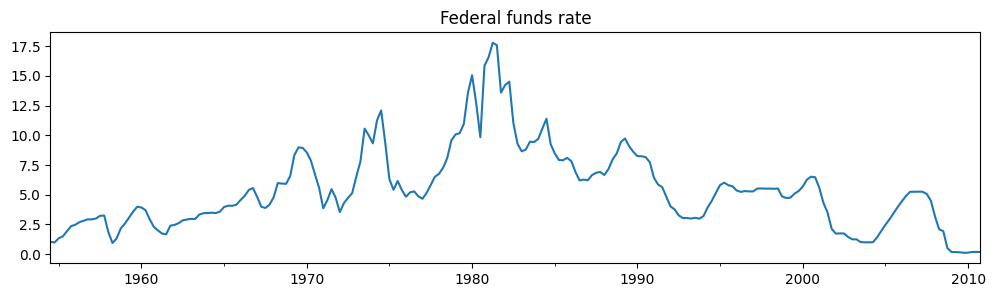:

res_fedfunds.summary()

:

Dep. Variable: No. Observations: y 226 MarkovRegression -508.636 Fri, 05 May 2023 1027.272 13:45:46 1044.375 07-01-1954 1034.174 - 10-01-2010 approx
coef std err z P>|z| [0.025 0.975] 3.7088 0.177 20.988 0.000 3.362 4.055
coef std err z P>|z| [0.025 0.975] 9.5568 0.300 31.857 0.000 8.969 10.145
coef std err z P>|z| [0.025 0.975] 4.4418 0.425 10.447 0.000 3.608 5.275
coef std err z P>|z| [0.025 0.975] 0.9821 0.010 94.443 0.000 0.962 1.002 0.0504 0.027 1.876 0.061 -0.002 0.103

Warnings:
 Covariance matrix calculated using numerical (complex-step) differentiation.

From the summary output, the mean federal funds rate in the first regime (the “low regime”) is estimated to be $$3.7$$ whereas in the “high regime” it is $$9.6$$. Below we plot the smoothed probabilities of being in the high regime. The model suggests that the 1980’s was a time-period in which a high federal funds rate existed.

:

res_fedfunds.smoothed_marginal_probabilities.plot(
title="Probability of being in the high regime", figsize=(12, 3)
)

:

<Axes: title={'center': 'Probability of being in the high regime'}>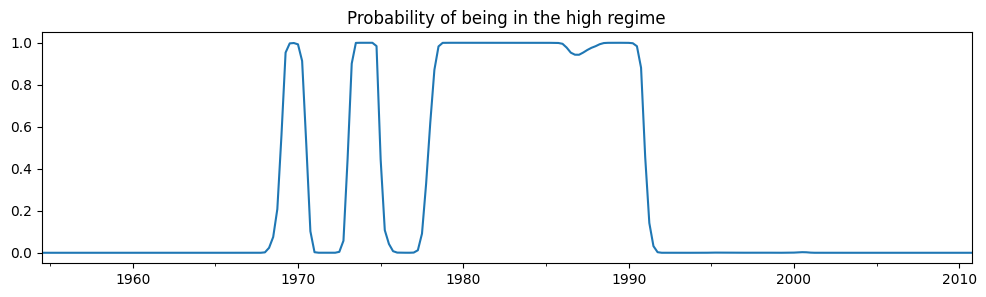From the estimated transition matrix we can calculate the expected duration of a low regime versus a high regime.

:

print(res_fedfunds.expected_durations)

[55.85400626 19.85506546]


A low regime is expected to persist for about fourteen years, whereas the high regime is expected to persist for only about five years.

## Federal funds rate with switching intercept and lagged dependent variable¶

The second example augments the previous model to include the lagged value of the federal funds rate.

$r_t = \mu_{S_t} + r_{t-1} \beta_{S_t} + \varepsilon_t \qquad \varepsilon_t \sim N(0, \sigma^2)$

where $$S_t \in \{0, 1\}$$, and the regime transitions according to

$\begin{split} P(S_t = s_t | S_{t-1} = s_{t-1}) = \begin{bmatrix} p_{00} & p_{10} \\ 1 - p_{00} & 1 - p_{10} \end{bmatrix}\end{split}$

We will estimate the parameters of this model by maximum likelihood: $$p_{00}, p_{10}, \mu_0, \mu_1, \beta_0, \beta_1, \sigma^2$$.

:

# Fit the model
mod_fedfunds2 = sm.tsa.MarkovRegression(
dta_fedfunds.iloc[1:], k_regimes=2, exog=dta_fedfunds.iloc[:-1]
)
res_fedfunds2 = mod_fedfunds2.fit()

:

res_fedfunds2.summary()

:

Dep. Variable: No. Observations: y 225 MarkovRegression -264.711 Fri, 05 May 2023 543.421 13:45:46 567.334 10-01-1954 553.073 - 10-01-2010 approx
coef std err z P>|z| [0.025 0.975] 0.7245 0.289 2.510 0.012 0.159 1.290 0.7631 0.034 22.629 0.000 0.697 0.829
coef std err z P>|z| [0.025 0.975] -0.0989 0.118 -0.835 0.404 -0.331 0.133 1.0612 0.019 57.351 0.000 1.025 1.097
coef std err z P>|z| [0.025 0.975] 0.4783 0.050 9.642 0.000 0.381 0.576
coef std err z P>|z| [0.025 0.975] 0.6378 0.120 5.304 0.000 0.402 0.874 0.1306 0.050 2.634 0.008 0.033 0.228

Warnings:
 Covariance matrix calculated using numerical (complex-step) differentiation.

There are several things to notice from the summary output:

1. The information criteria have decreased substantially, indicating that this model has a better fit than the previous model.

2. The interpretation of the regimes, in terms of the intercept, have switched. Now the first regime has the higher intercept and the second regime has a lower intercept.

Examining the smoothed probabilities of the high regime state, we now see quite a bit more variability.

:

res_fedfunds2.smoothed_marginal_probabilities.plot(
title="Probability of being in the high regime", figsize=(12, 3)
)

:

<Axes: title={'center': 'Probability of being in the high regime'}>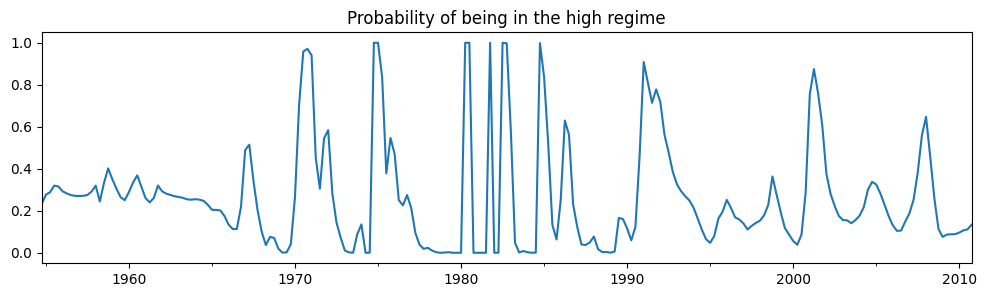Finally, the expected durations of each regime have decreased quite a bit.

:

print(res_fedfunds2.expected_durations)

[2.76105188 7.65529154]


## Taylor rule with 2 or 3 regimes¶

We now include two additional exogenous variables - a measure of the output gap and a measure of inflation - to estimate a switching Taylor-type rule with both 2 and 3 regimes to see which fits the data better.

Because the models can be often difficult to estimate, for the 3-regime model we employ a search over starting parameters to improve results, specifying 20 random search repetitions.

:

# Get the additional data
from statsmodels.tsa.regime_switching.tests.test_markov_regression import ogap, inf

dta_ogap = pd.Series(ogap, index=pd.date_range("1954-07-01", "2010-10-01", freq="QS"))
dta_inf = pd.Series(inf, index=pd.date_range("1954-07-01", "2010-10-01", freq="QS"))

exog = pd.concat((dta_fedfunds.shift(), dta_ogap, dta_inf), axis=1).iloc[4:]

# Fit the 2-regime model
mod_fedfunds3 = sm.tsa.MarkovRegression(dta_fedfunds.iloc[4:], k_regimes=2, exog=exog)
res_fedfunds3 = mod_fedfunds3.fit()

# Fit the 3-regime model
np.random.seed(12345)
mod_fedfunds4 = sm.tsa.MarkovRegression(dta_fedfunds.iloc[4:], k_regimes=3, exog=exog)
res_fedfunds4 = mod_fedfunds4.fit(search_reps=20)

:

res_fedfunds3.summary()

:

Dep. Variable: No. Observations: y 222 MarkovRegression -229.256 Fri, 05 May 2023 480.512 13:45:50 517.942 07-01-1955 495.624 - 10-01-2010 approx
coef std err z P>|z| [0.025 0.975] 0.6555 0.137 4.771 0.000 0.386 0.925 0.8314 0.033 24.951 0.000 0.766 0.897 0.1355 0.029 4.609 0.000 0.078 0.193 -0.0274 0.041 -0.671 0.502 -0.107 0.053
coef std err z P>|z| [0.025 0.975] -0.0945 0.128 -0.739 0.460 -0.345 0.156 0.9293 0.027 34.309 0.000 0.876 0.982 0.0343 0.024 1.429 0.153 -0.013 0.081 0.2125 0.030 7.147 0.000 0.154 0.271
coef std err z P>|z| [0.025 0.975] 0.3323 0.035 9.526 0.000 0.264 0.401
coef std err z P>|z| [0.025 0.975] 0.7279 0.093 7.828 0.000 0.546 0.910 0.2115 0.064 3.298 0.001 0.086 0.337

Warnings:
 Covariance matrix calculated using numerical (complex-step) differentiation.
:

res_fedfunds4.summary()

:

Dep. Variable: No. Observations: y 222 MarkovRegression -180.806 Fri, 05 May 2023 399.611 13:45:50 464.262 07-01-1955 425.713 - 10-01-2010 approx
coef std err z P>|z| [0.025 0.975] -1.0250 0.290 -3.531 0.000 -1.594 -0.456 0.3277 0.086 3.812 0.000 0.159 0.496 0.2036 0.049 4.152 0.000 0.107 0.300 1.1381 0.081 13.977 0.000 0.978 1.298
coef std err z P>|z| [0.025 0.975] -0.0259 0.087 -0.298 0.765 -0.196 0.144 0.9737 0.019 50.265 0.000 0.936 1.012 0.0341 0.017 2.030 0.042 0.001 0.067 0.1215 0.022 5.606 0.000 0.079 0.164
coef std err z P>|z| [0.025 0.975] 0.7346 0.130 5.632 0.000 0.479 0.990 0.8436 0.024 35.198 0.000 0.797 0.891 0.1633 0.025 6.515 0.000 0.114 0.212 -0.0499 0.027 -1.835 0.067 -0.103 0.003
coef std err z P>|z| [0.025 0.975] 0.1660 0.018 9.240 0.000 0.131 0.201
coef std err z P>|z| [0.025 0.975] 0.7214 0.117 6.177 0.000 0.493 0.950 4.001e-08 nan nan nan nan nan 0.0783 0.038 2.079 0.038 0.004 0.152 0.1044 0.095 1.103 0.270 -0.081 0.290 0.8259 0.054 15.208 0.000 0.719 0.932 0.2288 0.073 3.150 0.002 0.086 0.371

Warnings:
 Covariance matrix calculated using numerical (complex-step) differentiation.

Due to lower information criteria, we might prefer the 3-state model, with an interpretation of low-, medium-, and high-interest rate regimes. The smoothed probabilities of each regime are plotted below.

:

fig, axes = plt.subplots(3, figsize=(10, 7))

ax = axes
ax.plot(res_fedfunds4.smoothed_marginal_probabilities)
ax.set(title="Smoothed probability of a low-interest rate regime")

ax = axes
ax.plot(res_fedfunds4.smoothed_marginal_probabilities)
ax.set(title="Smoothed probability of a medium-interest rate regime")

ax = axes
ax.plot(res_fedfunds4.smoothed_marginal_probabilities)
ax.set(title="Smoothed probability of a high-interest rate regime")

fig.tight_layout()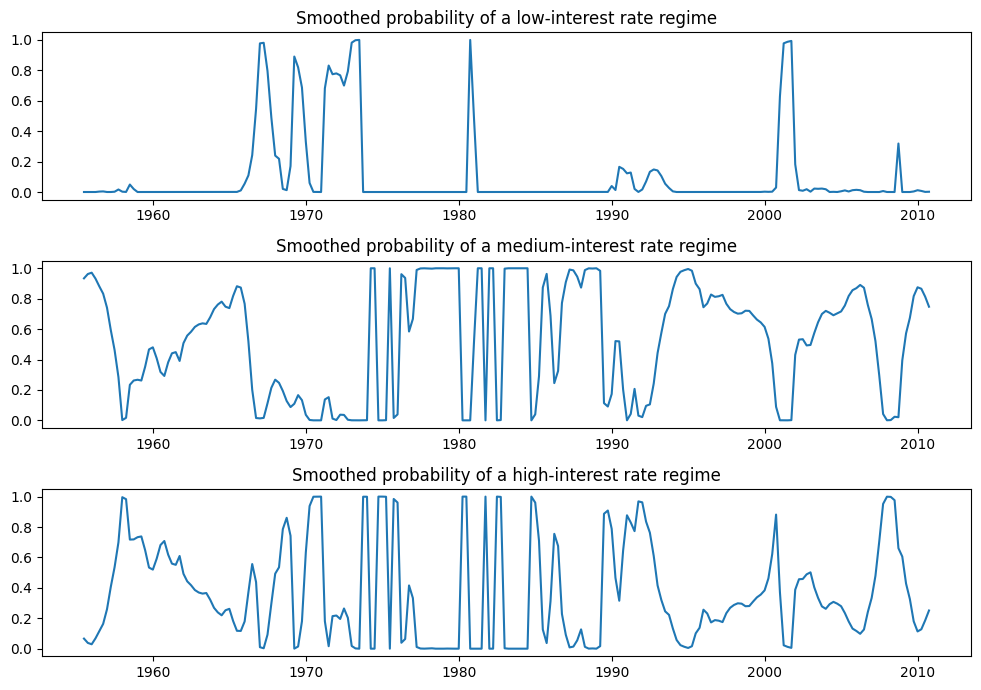## Switching variances¶

We can also accommodate switching variances. In particular, we consider the model

$y_t = \mu_{S_t} + y_{t-1} \beta_{S_t} + \varepsilon_t \quad \varepsilon_t \sim N(0, \sigma_{S_t}^2)$

We use maximum likelihood to estimate the parameters of this model: $$p_{00}, p_{10}, \mu_0, \mu_1, \beta_0, \beta_1, \sigma_0^2, \sigma_1^2$$.

The application is to absolute returns on stocks, where the data can be found at https://www.stata-press.com/data/r14/snp500.

:

# Get the federal funds rate data
from statsmodels.tsa.regime_switching.tests.test_markov_regression import areturns

dta_areturns = pd.Series(
areturns, index=pd.date_range("2004-05-04", "2014-5-03", freq="W")
)

# Plot the data
dta_areturns.plot(title="Absolute returns, S&P500", figsize=(12, 3))

# Fit the model
mod_areturns = sm.tsa.MarkovRegression(
dta_areturns.iloc[1:],
k_regimes=2,
exog=dta_areturns.iloc[:-1],
switching_variance=True,
)
res_areturns = mod_areturns.fit()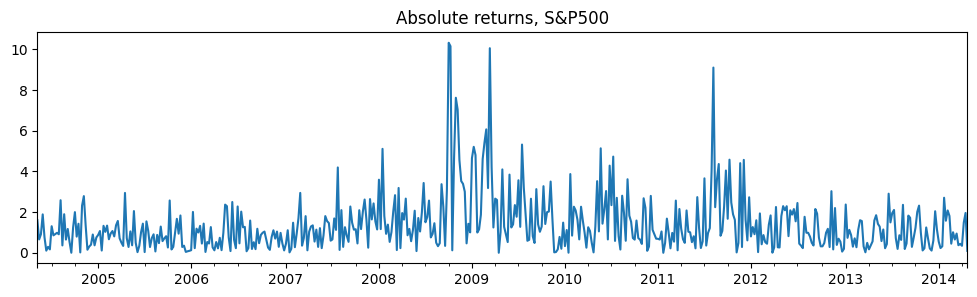:

res_areturns.summary()

:

Dep. Variable: No. Observations: y 520 MarkovRegression -745.798 Fri, 05 May 2023 1507.595 13:45:51 1541.626 05-16-2004 1520.926 - 04-27-2014 approx
coef std err z P>|z| [0.025 0.975] 0.7641 0.078 9.761 0.000 0.611 0.918 0.0791 0.030 2.620 0.009 0.020 0.138 0.3476 0.061 5.694 0.000 0.228 0.467
coef std err z P>|z| [0.025 0.975] 1.9728 0.278 7.086 0.000 1.427 2.518 0.5280 0.086 6.155 0.000 0.360 0.696 2.5771 0.405 6.357 0.000 1.783 3.372
coef std err z P>|z| [0.025 0.975] 0.7531 0.063 11.871 0.000 0.629 0.877 0.6825 0.066 10.301 0.000 0.553 0.812

Warnings:
 Covariance matrix calculated using numerical (complex-step) differentiation.

The first regime is a low-variance regime and the second regime is a high-variance regime. Below we plot the probabilities of being in the low-variance regime. Between 2008 and 2012 there does not appear to be a clear indication of one regime guiding the economy.

:

res_areturns.smoothed_marginal_probabilities.plot(
title="Probability of being in a low-variance regime", figsize=(12, 3)
)

:

<Axes: title={'center': 'Probability of being in a low-variance regime'}>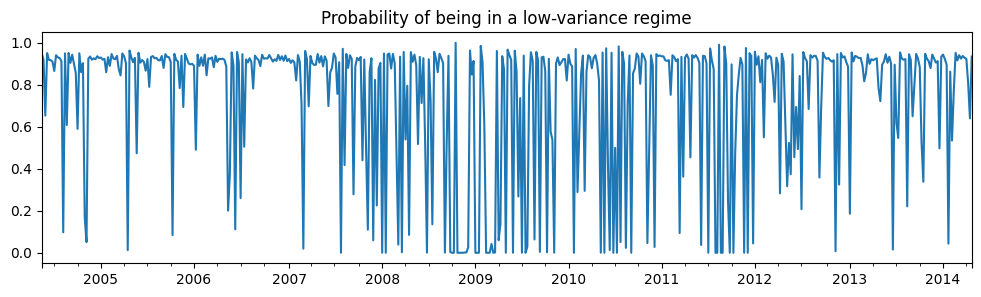Last update: May 05, 2023